Courses

RD Sharma Solutions - Ex - 4.2, Rational Numbers, Class 7, Math Notes | Study RD Sharma Solutions for Class 7 Mathematics - Class 7

Class 7: RD Sharma Solutions - Ex - 4.2, Rational Numbers, Class 7, Math Notes | Study RD Sharma Solutions for Class 7 Mathematics - Class 7

The document RD Sharma Solutions - Ex - 4.2, Rational Numbers, Class 7, Math Notes | Study RD Sharma Solutions for Class 7 Mathematics - Class 7 is a part of the Class 7 Course RD Sharma Solutions for Class 7 Mathematics.
All you need of Class 7 at this link: Class 7

Question 1:

Express each of the following as a rational number with positive denominator: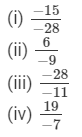Rational number with positive denominators:

(i) Multiplying the number by −-1, we get: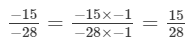(ii) Multiplying the number by −-1, we get: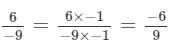(iii) Multiplying the number by −-1, we get: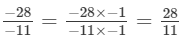(iv) Multiplying the number by −-1, we get: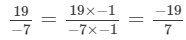Question 2:

Express 3/5 as a rational number with numerator:
(i) 6
(ii) −15
(iii) 21
(iv) −27

Rational number with numerator:

(i) 6 is: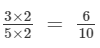(multiplying numerator and denominator by 2 )

(ii)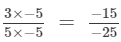(multiplying numerator and denominator by -5)

(iii)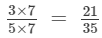(multiplying numerator and denominator by 7)

(iv)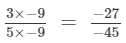(multiplying numerator and denominator by -9)

Question 3:

Express 5757 as a rational number with denominator:
(i) −14
(ii) 70
(iii) −28
(iv) −84

5/7 as a rational number with denominator:
(i) −14 is: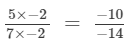(multiplying numerator and denominator by -2)

(ii) 70 is :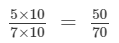(multiplying numerator and denominator by 10)

(iii) −28 is: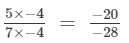(multiplying numerator and denominator by -4)

(iv) −84 is: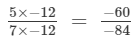(multiplying numerator and denominator by -12)

Question 4:

Express 3/4 as a rational number with denominator:
(i) 20
(ii) 36
(iii) 44
(iv) −80

3/4 as rational number with denominator:

(i)
20 is: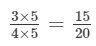(multiplying numerator and denominator by 5)

(ii)
36 is: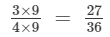(multiplying numerator and denominator by 9)

(iii)
44 is: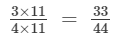(multiplying numerator and denominator by 11)

(iv)
−80 is: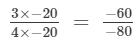(multiplying numerator and denominator by -20)

Question 5:

Express 2/5 as a rational number with numerator:
(i) −56
(ii) 154
(iii) −750
(iv) 500

2/5 as a rational number with numerator:

(i)
−56 is: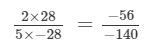(multiplying numerator and denominator by -28)

(ii)
154 is: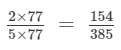(multiplying numerator and denominator by 77)

(iii)
−750 is: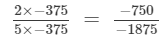(multiplying numerator and denominator by -375)

(iv)
500 is: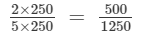(multiplying numerator and denominator by 250)

Question 6:

Express −192/108 as a rational number with numerator:
(i) 64
(ii) −16
(iii) 32
(iv) −48

Rational number with numerator:

(i)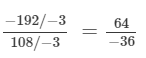(Dividing the numerator and denomintor by −3)

(ii)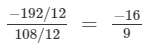(Dividing the numerator and denomintor by 12)

(iii)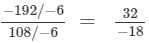(Dividing the numerator and denomintor by −6)

(iv)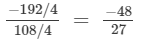(Dividing the numerator and denomintor by 4)

Question 7:

Express 168 / −294 as a rational number with denominator:
(i) 14
(ii) −7
(iii) −49
(iv) 1470

Rational number with denominator:

(i)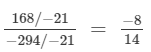(Dividing the numerator and denomintor by −21)

(ii)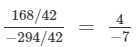(Dividing the numerator and denomintor by 42)

(iii)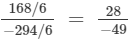(Dividing the numerator and denomintor by 6)

(iv)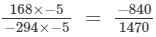(Dividing the numerator and denomintor by −5)

Question 8:

Write −14/42 in a form so that the numerator is equal to:
(i) −2
(ii) 7
(iii) 42
(iv) −70

Rational number with numerator:

(i)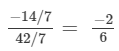( Dividing numerator and denominator by 7)

(ii)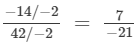( Dividing numerator and denominator by -2)

(iii)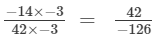( Dividing numerator and denominator by -3)

(iv)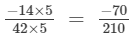( Dividing numerator and denominator by 5)

Question 9:

Select those rational numbers which can be written as a rational number with numerator 6: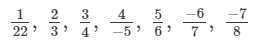Given rational numbers that can be written as a rational number with numerator 6 are:

1/22 (On multiplying by 6) = 6/132

2/3 (On multiplying by 3) = 6/9

3/4 (On multiplying by 2) = 6/8

−6/7 (On multiplying by −1) = 6/−7

Question 10:

Select those rational numbers which can be written as a rational number with denominator 4: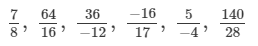Given rational numbers that can be written as a rational number with denominator 4 are:

7/8 (On dividing by 2) = 3.5/4

64/16 (On dividing by 4) =16/4

36/−12(On dividing by 3) =12/−4 = −12/4

−16/17 can't be expressed with a denominator 4.

5/−4(On multiplying by −1) =−5/4

140/28(On dividing by 7) =20/4

Question 11:

In each of the following, find an equivalent form of the rational number having a common denominator: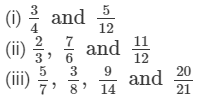Equivalent forms of the rational number having common denominator are: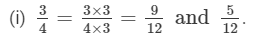(ii)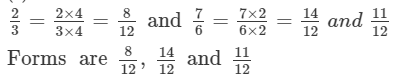(iii)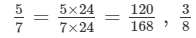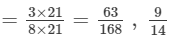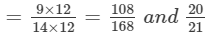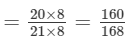Forms are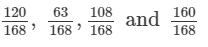The document RD Sharma Solutions - Ex - 4.2, Rational Numbers, Class 7, Math Notes | Study RD Sharma Solutions for Class 7 Mathematics - Class 7 is a part of the Class 7 Course RD Sharma Solutions for Class 7 Mathematics.
All you need of Class 7 at this link: Class 7Use Code STAYHOME200 and get INR 200 additional OFF Use Coupon Code

Top Courses for Class 7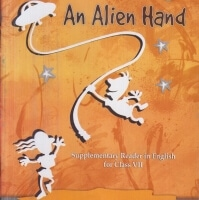RD Sharma Solutions for Class 7 Mathematics

97 docs

Top Courses for Class 7Track your progress, build streaks, highlight & save important lessons and more!

,

,

,

,

,

,

,

,

,

,

,

,

,

,

,

,

,

,

,

,

,

,

,

,

,

,

,

,

,

,

;